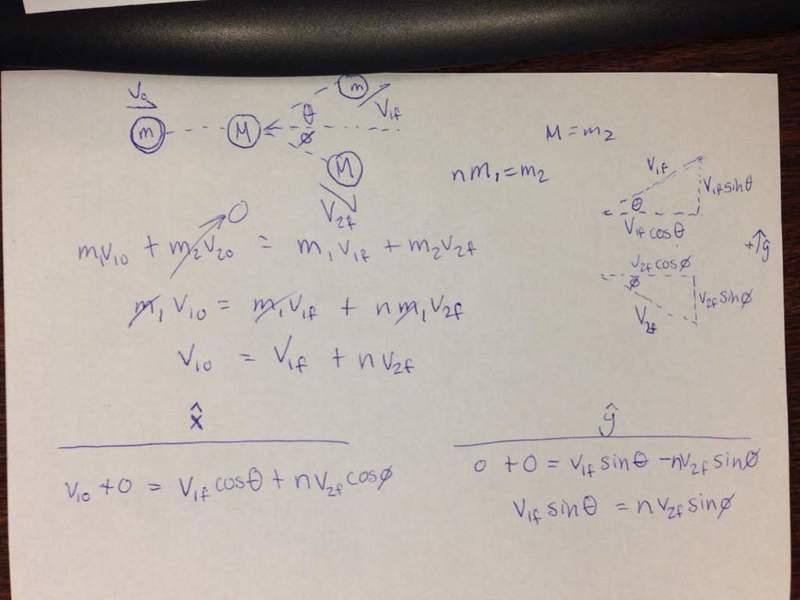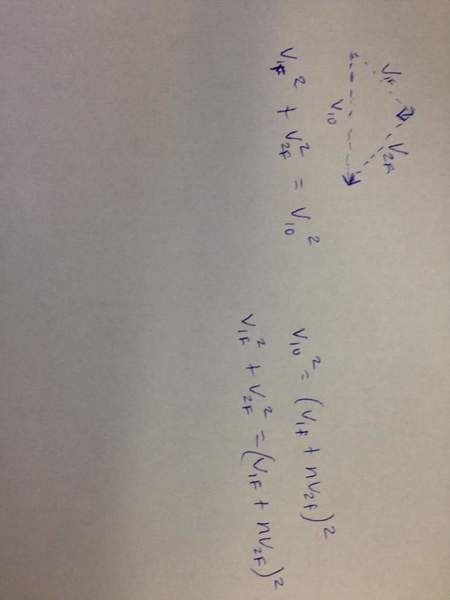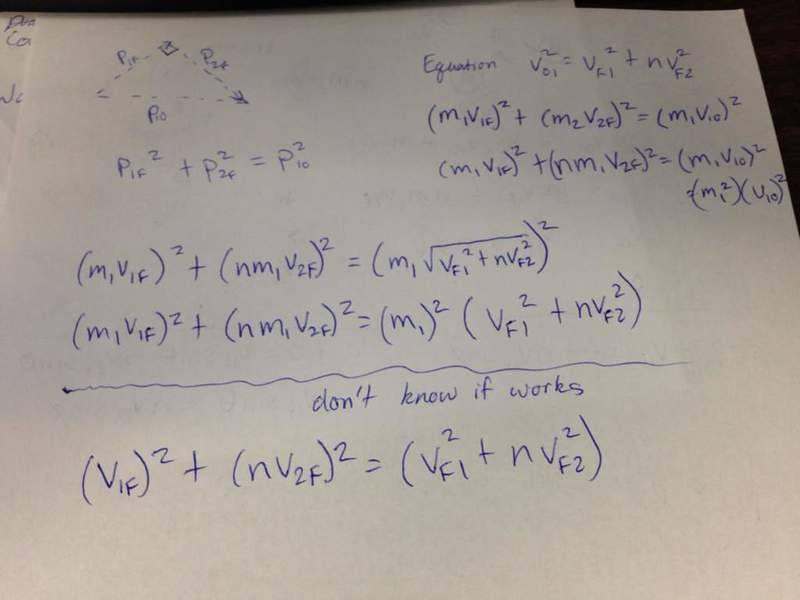# Glancing Elastic Collison

Fizics14
1. Two masses, m and M are involved in a glacing collision as seen below where θ and ø= pi/2.
If M = nm what must n be such that the collision is elastic?

Remember if θ+ø=pi/2 then cos(θ)=sin(ø) and cos(ø)=sin(θ)

2. I am suppose to find an number for n.

3. ∑KEo=∑KEf
1/2m$_{1}$v$^{2}_{0}$+0=1/2m$_{1}$v$^{2}_{f1}$+1/2m$_{2}$v$^{2}_{f2}$

substitute m$_{1}$n for m$_{2}$ and cancel the 1/2m$_{1}$

v$^{2}_{o1}$=v$^{2}_{f1}$+nv$^{2}_{f2}$
n=$\frac{v^{2}_{o1}-v^{2}_{f1}}{v^{2}_{f2}}$

Not sure what to do from here please help. I know I'm probably suppose to use the θ and ø, but I'm not sure how to incorporate it.

## Homework Statement

Known: M=nm, θ+ø=pi/2
Unknown: n

## Homework Equations

W$_{NC}$=ΔKE+ΔPE
KE=$\frac{1}{2}$mv$^{2}$
Momentum=∑p=mv$_{f}$-mv$_{o}$

## The Attempt at a Solution

PS sorry I kinda messed this up it is my first post.

Last edited:

## Answers and Replies

Homework Helper
1. Two masses, m and M are involved in a glacing collision as seen below where θ and ø= pi/2.
If M = nm what must n be such that the collision is elastic?

Remember if θ+ø=pi/2 then cos(θ)=sin(ø) and cos(ø)=sin(θ)

2. I am suppose to find an number for n.

3. ∑KEo=∑KEf
1/2m$_{1}$v$^{2}_{0}$+0=1/2m$_{1}$v$^{2}_{f1}$+1/2m$_{2}$v$^{2}_{f2}$

substitute m$_{1}$n for m$_{2}$ and cancel the 1/2m$_{1}$

v$^{2}_{o1}$=v$^{2}_{f1}$+nv$^{2}_{f2}$
n=$\frac{v^{2}_{o1}-v^{2}_{f1}}{v^{2}_{f2}}$

Not sure what to do from here please help. I know I'm probably suppose to use the θ and ø, but I'm not sure how to incorporate it.

## Homework Statement

Known: M=nm, θ+ø=pi/2
Unknown: n

## Homework Equations

W$_{NC}$=ΔKE+ΔPE
KE=$\frac{1}{2}$mv$^{2}$
Momentum=∑p=mv$_{f}$-mv$_{o}$

## The Attempt at a Solution

PS sorry I kinda messed this up it is my first post.
From conservation of energy:

mv02 = nmv12 + mv22

which can be rewritten:

(1) $v_0^2 = nv_1^2 + v_2^2$

Now draw the vectors for the initial and final momenta of each body. What is the relationship between the initial total momentum and the final total momentum?

Since the final momentum vectors are at right angles to each other, what is the length of the sum of those two vectors (i.e. the magnitude of the final total momentum)? How is that length related to the length of the initial momentum vector?

Now compare that relationship with (1).

AM

Fizics14
The momentum should be equal since it is elastic so I'm assuming the vector before the collision should be equal to the vectors after the collision right? So I solved for two different equations. Separated them by x and y components. I'm not sure what to solve for now. I really need help I have to present this to the class on Monday!dauto
The momentum should be equal since it is elastic so I'm assuming the vector before the collision should be equal to the vectors after the collision right? So I solved for two different equations. Separated them by x and y components. I'm not sure what to solve for now. I really need help I have to present this to the class on Monday!
View attachment 68814

while that method will work, it is easier to follow Andrew Mason's advice and draw the diagram representing the addition of the two vectors (the momenta of the two objects after collision). Every diagram representing the addition of two vectors is a triangle. For this problem that triangle turns out to be a square triangle which allows you to use Pythagoras theorem to relate the momenta of the particles before and after the collision.

Fizics14
How does finding that vector help me solve for n?

dauto
It will give you another equation. right now you have only one equation $v^{2}_{o1}=v^{2}_{f1}+nv^{2}_{f2},$ but that isn't enough because you don't know the speeds.

Fizics14
It will give you another equation. right now you have only one equation $v^{2}_{o1}=v^{2}_{f1}+nv^{2}_{f2},$ but that isn't enough because you don't know the speeds.

Okay so is this correct? Can you square both sides of that equation?
Here is my attempt and now I'm stuck...dauto
Okay so is this correct? Can you square both sides of that equation?
Here is my attempt and now I'm stuck...
View attachment 68815

You're almost there. Your triangle looks right, but you labeled it with speeds v. speed isn't a conserved quantity. you should've labeled the triangle with momenta p where p = mv. Momentum is a conserved quantity.

Fizics14
When I change it to momentum I think it just solves for the equation we already have right?
By the way thank you so much for helping!!! I'm sorry I'm so bad at this :/

Last edited:
dauto
When I change it to momentum I think it just solves for the equation we already have right?
By the way thank you so much for helping!!!

No, not right. You get a different equation. Do it.

Fizics14
My attempt:dauto
Yes, that's the equation. Now, what can be canceled out of that equation at the bottom of the page?

Homework Helper
My attempt:
View attachment 68818
Ok. So what value of n satisfies that relationship:

$v_{f1}^2 + (nv_{f2})^2 = (v_{f1}^2 + nv_{f2}^2)$

AM

Last edited:
Fizics14
Is it just 1?

dauto
ding ding ding!!!

Fizics14
Sweet haha thanks you guys so much!

Homework Helper
Is it just 1?

Very good. Is that a guess? Work it out algebraically to show that n = 1 and then we will give you full marks.

AM

dauto
There is another solution. Can you see it?

Fizics14
I'm guessing zero so I should probably put both

dauto
Yes, it is zero, but don't guess it. Find it algebraically. Even though zero is a solution, there is something wrong with it. Can you tell what it is?

Fizics14
Yes I did find it algebraically, but there is something wrong because then the mass would just be zero which cant be true.

dauto
Well there are particles that are truly massless such as the photon but they are governed by relativistic formulae which are different than the ones you used, so you would have to redo your calculations. Alternatively you might say that the particle really isn't massless but has a negligible mass, in which case the angle really isn't 90 degrees, but it is close.

Last edited:
Fizics14
I was thinking of a point particle with infinitesimal mass like that but I don't think that's what the problem is about. Thank you! I'll be back no doubt!

Homework Helper
Well there are particles that are truly massless such as the photon but they are governed by relativistic formulae which are different than the ones you used, so you would have to redo your calculations. Alternatively you might say that the particle really isn't massless but has a negligible mass, in which case the angle really isn't 90 degrees, but it is close.

How does n=0 satisfy:

$v_{f1}^2 + nv_{f2}^2 = v_{f1}^2 + v_{f2}^2$?

If n is very small it does not begin to satisfy the relationship. I think n=1 is the only solution.

By the way, billiards players know that the angle between the cue ball and object ball is 90° (absent spin on the cue ball).

AM

dauto
How does n=0 satisfy:

$v_{f1}^2 + nv_{f2}^2 = v_{f1}^2 + v_{f2}^2$?

If n is very small it does not begin to satisfy the relationship. I think n=1 is the only solution.

By the way, billiards players know that the angle between the cue ball and object ball is 90° (absent spin on the cue ball).

AM

that's not the equation I got. My solution looks like

$v_{f1}^2 + nv_{f2}^2 = v_{f1}^2 + n^2v_{f2}^2$

Homework Helper
that's not the equation I got. My solution looks like

$v_{f1}^2 + nv_{f2}^2 = v_{f1}^2 + n^2v_{f2}^2$
Ok. Same thing. There is really a discontinuity at n=0. For any n in an arbitrarily small neighbourhood of 0, n does not come close to satisfying the equation.

The condition that the bodies recoil at 90° to each other is not met using classical mechanics if the mass of the second particle (the one initially at rest) is zero.

AM

Last edited: